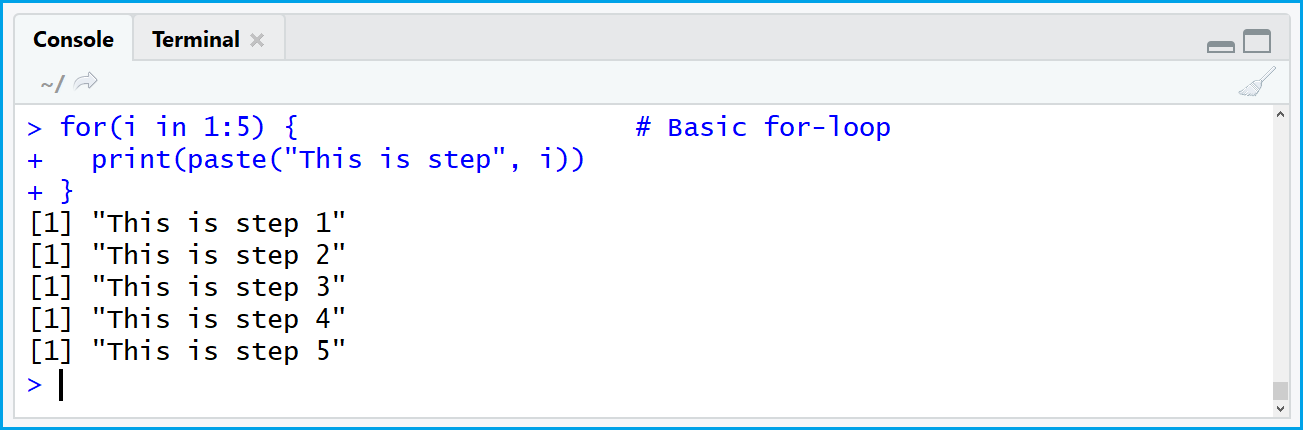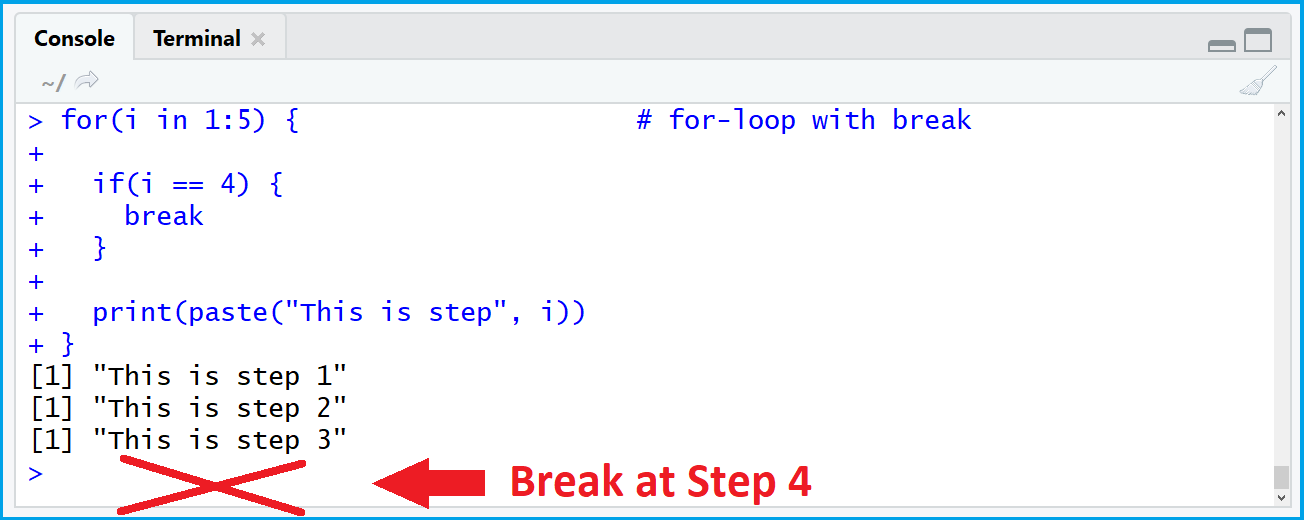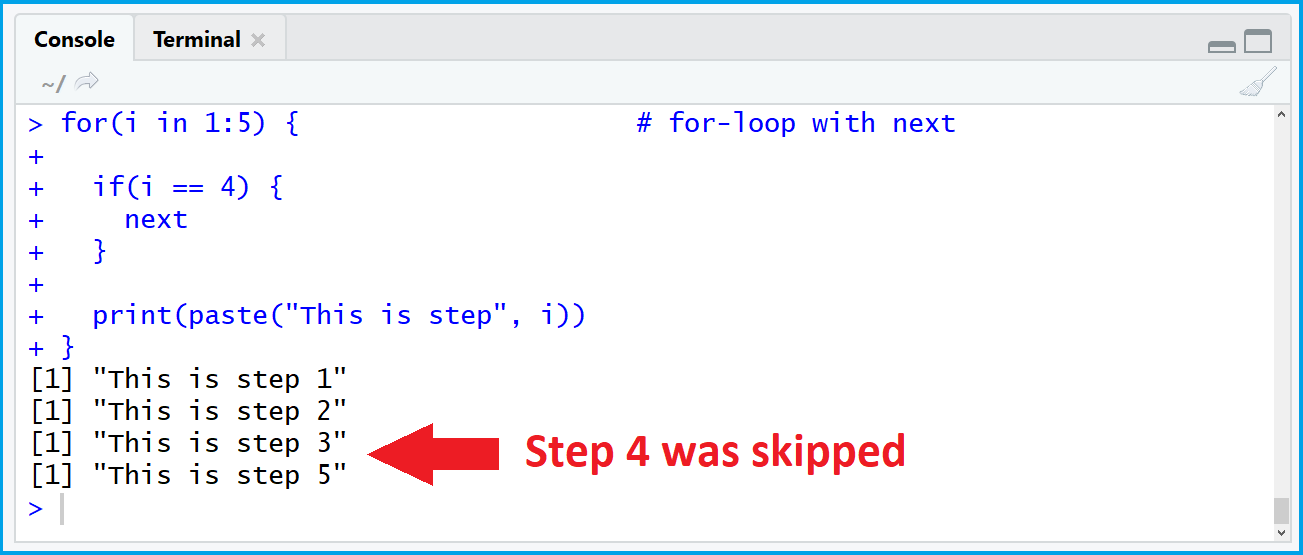# break & next Functions in R for-loop (2 Examples)

This tutorial shows how to use the break and next commands within a for-loop in R.

Without further ado, let’s move directly to the examples!

## Create Basic for-loop in R (without break or next)

In the examples of this tutorial, I’ll use the following for-loop as basement:

```for(i in 1:5) { # Basic for-loop print(paste("This is step", i)) }```Figure 1: Basic Syntax of for-loop in R.

As you can see based on the previous figure, our example for-loop prints the words “This is step” and the running index i to the RStudio console.

Our loop runs from 1 to 5 and returns therefore five sentences. Let’s see what happens when we use break and next…

## Example 1: break within for-loop

We can insert a break in our for-loop as shown in the following R code:

```for(i in 1:5) { # for-loop with break   if(i == 4) { break }   print(paste("This is step", i)) }```Figure 2: for-loop with break Function.

As shown in Figure 2, the loop stops (or “breaks”) when our running index i is equal to the value 4. For that reason, R returns only three sentences.

## Example 2: next within for-loop

The next statement can be useful, in case we want to continue our loop after a certain break. The following R code skips step 4 of our loop, but continues again afterwards:

```for(i in 1:5) { # for-loop with next   if(i == 4) { next }   print(paste("This is step", i)) }```Figure 3: for-loop with next Function.

Figure 3 shows the output after inserting the next function into our for-loop. R printed all steps beside step 4.

Note: The codes of the previous examples can also be applied to other types of loops (e.g. while loops).

## Further Resources for the Handling of Loops

In addition, you can have a look at the other R tutorials on my website:

This article explained how to apply break and next in the R programming language. Leave me a comment below in case you have any further questions.